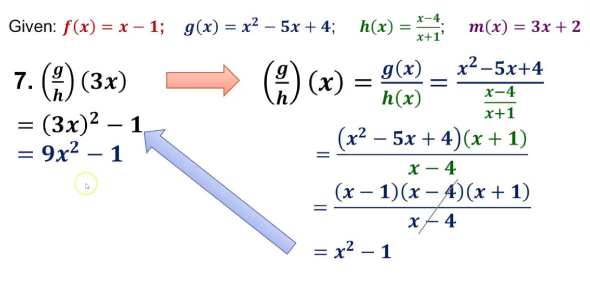# General Mathematics Quiz

13 Questions | Total Attempts: 1126SettingsTake this ultimate quiz on general knowledge math. It's pretty difficult so you might need extra time to score a perfect.

Related Topics
• 1.
What'd be x if three angles of a triangle are x, 2x and 3x degrees?
• A.

45

• B.

30

• C.

60

• D.

25

• 2.
What'd be x and y if, 5x + 3y = 11 and 2x - 7y = -12?
• A.

1 and 2

• B.

Both -1

• C.

2 and 1

• D.

-1 and 2

• 3.
If xy is a 2-digit no. & x+y = 7; it becomes yx if 27 is added; what is xy?
• A.

43

• B.

61

• C.

25

• D.

34

• 4.
If DY = 42, GV = 75, and BZ = 21 then what is FT?
• A.

81

• B.

79

• C.

45

• D.

67

• 5.
Paul completes 7 surveys in 8 days. How many can he complete in 40 days?
• A.

35

• B.

55

• C.

42

• D.

48

• 6.
What is the missing number in the series?: 0, 7, 26, 63, 124, ?, 342
• A.

215

• B.

255

• C.

235

• D.

311

• 7.
The diagonals of a rhombus bisect each other at what angles?
• A.

Acute

• B.

Right

• C.

Obtuse

• D.

Straight

• 8.
If 2/3 of x is 60, then what is 4/5 of x?
• A.

85

• B.

55

• C.

68

• D.

72

• 9.
Male:Female population in a town of 1500 is 3:2; Number of men in the town?
• A.

900

• B.

500

• C.

750

• D.

1000

• 10.
What is -40 degrees Fahrenheit in Celsius scale?
• A.

40 Degrees

• B.

75 Degrees

• C.

-40 Degrees

• D.

33 Degrees

• 11.
Which of these halogens has the largest electron affinity?
• A.

Bromine

• B.

Chlorine

• C.

Fluorine

• D.

Iodine

• 12.
What is the value of 1 ≈ 10 ≈ 100 ≈ 1000?
• A.

0.0001

• B.

1.0E-6

• C.

1.0E-5

• D.

1.0E-8

• 13.
X is 80% of y; then y is how many percent of x?
• A.

100%

• B.

125%

• C.

122%

• D.

75%